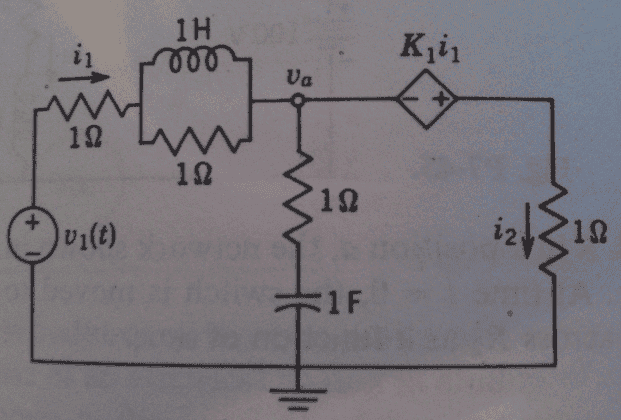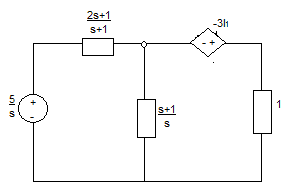# Circuit with dependent voltage source

• Engineering
magnifik
I am trying to solve for va in the circuit below, which has a current controlled voltage source:Given: v1 = 5u(t), K1 = -3, the circuit is not energized at t = 0

I began by writing loop equations for each of the three parts of the circuit. Then I realized I am not quite sure how to get va from the currents. I am wondering is there a better way to approach this problem? Or if I continue with the method I started with, how do I solve for va with the currents?

Homework Helper
Gold Member
You can write just 1 equation with 1 unknown, Va, or 2 equations with 2 unknowns, Va and i2. The second approach is a bit safer. The result is exactly the same.

Just sum currents at node Va.

magnifik
You can write just 1 equation with 1 unknown, Va, or 2 equations with 2 unknowns, Va and i2. The second approach is a bit safer. The result is exactly the same.

Just sum currents at node Va.

at node va is it correct that i1 = i2
i am still a little confused. after i solve for the currents (there are three of them in my method), how do i relate this to va?

Homework Helper
Gold Member
No. What about the current going thru the 1 ohm & the 1F? i1 = that current plus i2.

OK I'll give it to you: i1 = Va/Z + i2. Now, what is Z?

magnifik
No. What about the current going thru the 1 ohm & the 1F? i1 = that current plus i2.

OK I'll give it to you: i1 = Va/Z + i2. Now, what is Z?

Z is for the 1Ω resistor and 1F capacitor

Homework Helper
Gold Member
Absolutely right. What is Z(s)?

magnifik
Absolutely right. What is Z(s)?

Z(s) = (s+1)/s

Homework Helper
Gold Member
Correct. What is i1(V1, Va)?

magnifik
Correct. What is i1(V1, Va)?

(va - v1) / (1 + (s || 1)) + va/((s+1)/s) ?
i1 = (va - v1)/1

Homework Helper
Gold Member
(va - v1) / (1 + (s || 1)) + va/((s+1)/s) ?
i1 = (va - v1)/1

Your first equation: since i1 = -(Va - V1)/Z1, it can't be right, can it?
where Z1 = 1 + s||1

Your 2nd equation: how on Earth did you get that?

magnifik
Your first equation: since i1 = -(Va - V1)/Z1, it can't be right, can it?
where Z1 = 1 + s||1

Your 2nd equation: how on Earth did you get that?

i thought i1 was the current going through the resistor only, but then i realized it also goes through the parallel inductor/resistor. or is i1 = v1/R ? yup I'm confused. i meant to set va-v1/Z1 + va/((s+1)/s) = 0, but i am still failing to see the connection to i1

Homework Helper
Gold Member
First, get i1(V1,Va) right. Until you get that right and understand it we can't go on.

Then: remember a ways back I gave you
i1 = Va/Z + i2?

What about i2? Look at the voltage across the right-hand 1 ohm, what is the voltage there?

magnifik
First, get i1(V1,Va) right. Until you get that right and understand it we can't go on.

Then: remember a ways back I gave you
i1 = Va/Z + i2?

What about i2? Look at the voltage across the right-hand 1 ohm, what is the voltage there?

can i just do what i did originally - solve for the currents in each of the three loops. then use i1 = Va/Z + i2 to get the final answer?

my three loop equations were:
for the left loop
5 = 3ia - ib - ic + ∫(ia - ic) dt
for the top loop
0 = ib - ia + dib/dt
for the right loop
-3ia = 2ic - ia + ∫(ic - ia) dt

Homework Helper
Gold Member
I'm real surprised you are using diff. eq's instead of Laplace. Are you supposed to do it purely using d.e.'s or do you go from the d.e.'s to the Laplace? BTW that's a good way to do it.

If you will tell me what ia, ib and ic are I can look further at it. I'm having a tough time trying to second-guess the loops. Please label the resistors R1, R2, R3 and R4 from left to right, and use L and C. Thanks.

In case you've lost interest by now: if your loop equations are correct, the answer is yes.

Last edited:
magnifik
I'm real surprised you are using diff. eq's instead of Laplace. Are you supposed to do it purely using d.e.'s or do you go from the d.e.'s to the Laplace? BTW that's a good way to do it.

If you will tell me what ia, ib and ic are I can look further at it. I'm having a tough time trying to second-guess the loops. Please label the resistors R1, R2, R3 and R4 from left to right, and use L and C. Thanks.

In case you've lost interest by now: if your loop equations are correct, the answer is yes.

I start with diff eqs then convert to laplace. I will put my final answer once I do the work to find it.

EDIT: In my equations, i1 = ia, i2 = ic, ib is the loop current for the inductor/resistor in parallel

Last edited:
Homework Helper
Gold Member
Just a tip, if the network is initially inactive (no capacitor charge or inductor current) then it's usually more straight-forward to just treat the components as Z(s) or Y(s).

For example, commponents in parallel are much more easily handled as admittances [ Y(s) ] than impedances. E.g. a capacitor and resistor in parallel is Y = G + sC where G = 1/R.

A voltage divider is equally well written as Y2/(Y1 + Y2) as Z1/(Z1 + Z2).

Etc. Idea is to make life as simple as possible. Avoid more mistakes that way too.

OK I'll see what you come up with.

magnifik
Just a tip, if the network is initially inactive (no capacitor charge or inductor current) then it's usually more straight-forward to just treat the components as Z(s) or Y(s).

For example, commponents in parallel are much more easily handled as admittances [ Y(s) ] than impedances. E.g. a capacitor and resistor in parallel is Y = G + sC where G = 1/R.

A voltage divider is equally well written as Y2/(Y1 + Y2) as Z1/(Z1 + Z2).

Etc. Idea is to make life as simple as possible. Avoid more mistakes that way too.

OK I'll see what you come up with.

I'm trying to solve using your advice, though I used impedance rather than admittance. Is this a correct simplification?Homework Helper
Gold Member
Excellent! You're on your way. Now let's see you handle the current-controlled voltage source, and remember K = -3, not +3.

[Here's what I meant about Y vs. Z: the way I got the parallel impedance of the L and R is as follows: Y = G + 1/sL = (sLG+1)/sL where G = 1/R.

Z = 1/Y = sL/(sLG + 1).

That's easier, for me at least, than 1/Z = 1/R + sL etc. If L had been a C it would have been even nicer to go Y rather than Z. Then, get Z = 1/Y at the end. Don't be afraid to keep G's running around in your expressions rather than R's if it makes the math go easier.]

Last edited: